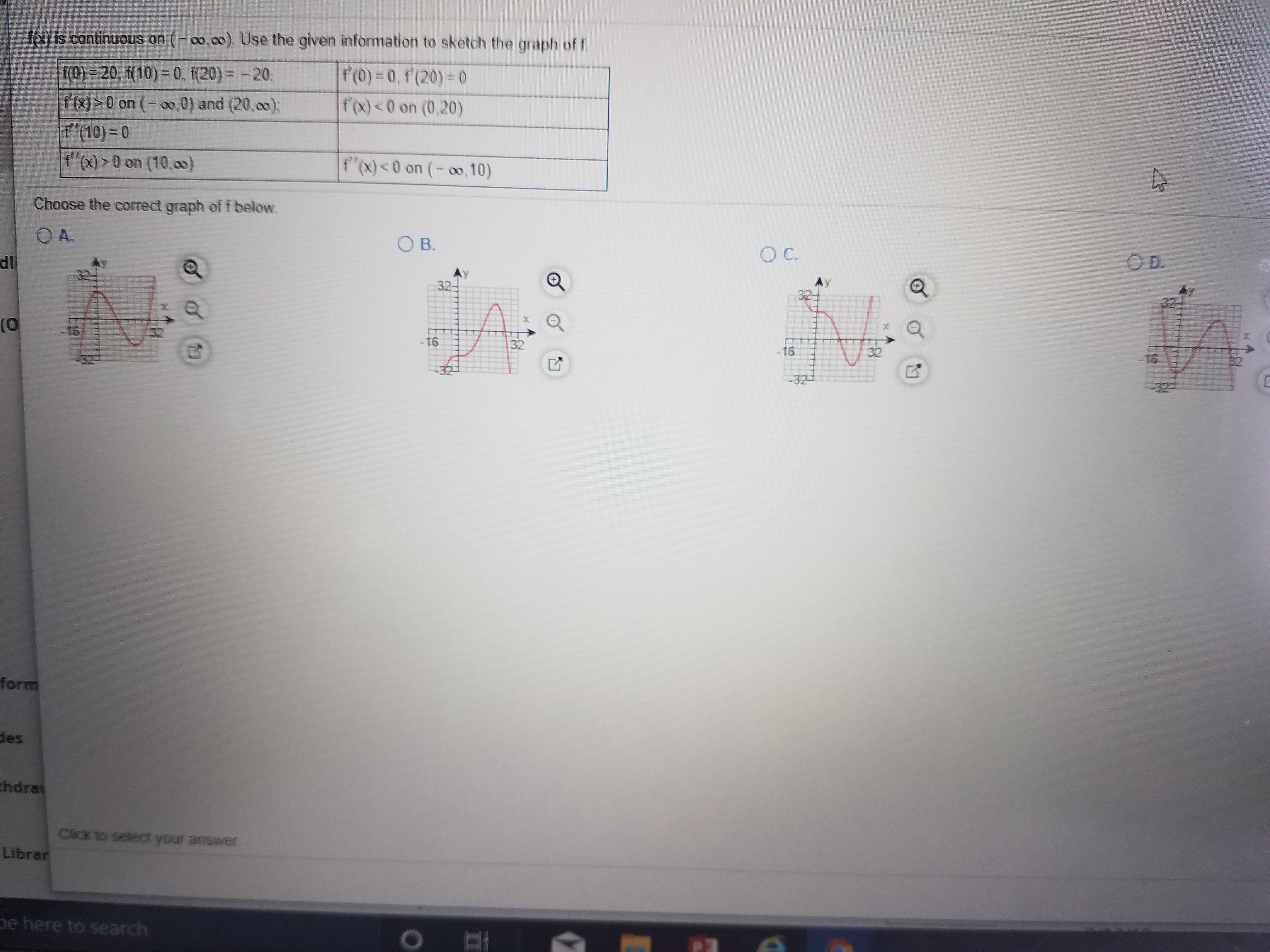# fo) is continuous on (- co,oo). Use the given information to sketch the graph of ff(0)-0, f (20) 0f'(x) 0 on (-oo,0) and (20,co);f'(10) = 0f'x)>0 on (10,00)f'(x)

Question
33 viewshelp_outlineImage Transcriptionclosefo) is continuous on (- co,oo). Use the given information to sketch the graph of f f(0)-0, f (20) 0 f'(x)<0 on (0,20) f(0) 20, f(10) 0, f(20) -20 f (x)> 0 on (-oo,0) and (20,co); f'(10) = 0 f'x)>0 on (10,00) f'(x)<0 on (-o,10) Choose the correct graph of f below. OA. O B. O C. OD. dli 32 (0 16 -16 32 32 -16 32 -321 form des chdra Click to select your answer Librar pe here to search fullscreen
check_circle

Step 1

f(0) = 20

Graphs in option B and D show f(0) to be negative. Hence, these two options are ruled out.

Step 2

f'(x) > 0 on (-infinity, 0)

This implies f(x) is an increasing function in the interval (-infinity, 0)

Exmine the behavior of the graph in option A and C in the range (-infinity, 0...

### Want to see the full answer?

See Solution

#### Want to see this answer and more?

Solutions are written by subject experts who are available 24/7. Questions are typically answered within 1 hour.*

See Solution
*Response times may vary by subject and question.
Tagged in

### Calculus